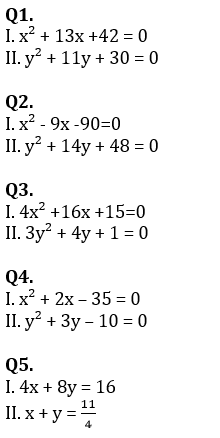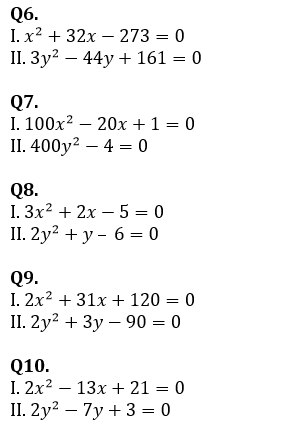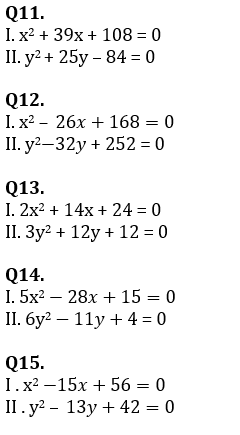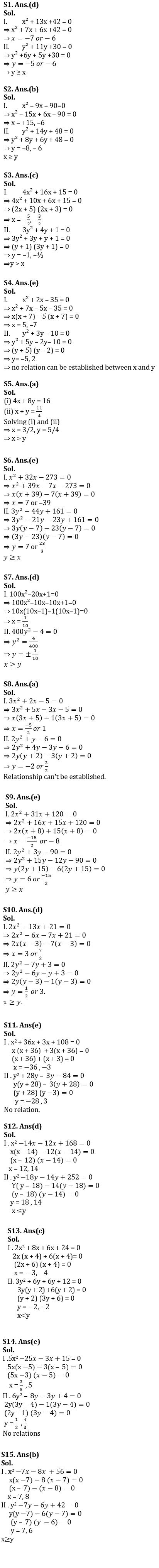# Quantitative Aptitude Quiz For SBI PO, Clerk Prelims 2021- 14th April

Directions (1-5): In each of these questions, two equations (I) and (II) are given. You have to solve both the equations and give answer
(a) if x > y
(b) if x ≥ y
(c) if x < y
(d) if x ≤ y
(e) if x = y or no relation can be established between x and y.Directions (6 – 10): In each question two equations numbered (I) and (II) are given. Student should solve both the equations and mark appropriate answer.
(a) If x = y or no relation can be established
(b) If x > y
(c) If x < y
(d) If x ≥ y
(e) If x ≤ yDirections (11-15): In each of these questions, two equations (I) and (II) are given. You have to solve both the equations and give answer
(a) if x > y
(b) if x ≥ y
(c) if x < y
(d)if x ≤ y
(e) if x = y or no relation can be established between x and y.Solutions×

Thank You, Your details have been submitted we will get back to you.
×
OR

×
OR